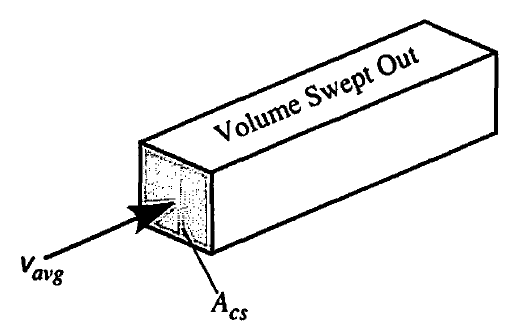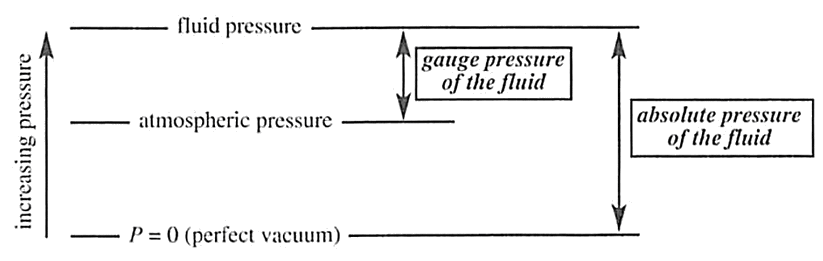# Fluids and Fluid Flow¶

A computer lets you make more mistakes faster than any invention in human history - with the possible exceptions of handguns and tequila.

## Fluids¶

We usually group fluids into gases and liquids.

Gases are characterized as loosely associated molecules that are normally not close together and that travel through space for long distances (many times larger than the molecular diameter) before colliding with each other. The velocity of their travel depends on the temperature of the gas.

Liquids are characterized by molecules that are very close together (on the same order as their molecular diameter) and that are in collision with each other very frequently as they move around each other. The velocity of that motion and the rate of that collision depend on the temperature of the liquid.

## Velocity¶

In most processes of interest, there will be movement of the fluid and a resulting velocity, pressure, shear stresses, …

The velocity of a fluid will depend on a number of factors, including the flow geometry, the fluid properties (density, viscosity), and the pressure.

### Velocity profiles¶

The velocity profile indicates the magnitude of the velocity as a function of position. This is analogous to a concentration profile.

In this course, a couple of geometries of interest are a flat plate and a tube or pipe.

A major feature of the velocity profile is the no slip condition at the surface. That is, the velocity goes to zero ($$v = 0$$) at the surface.

Laminar flow in a tube:Laminar represents one of the two classifications of flow; the other is turbulent, which has a different shape to its velocity profile.

### Boundary layers¶

A key aspect of flow across a surface is the formation of a boundary layer.

The boundary layer (hydrodynamic, concentration, or thermal) is a thin layer very close to then surface in which significant changes in velocity, concentration, and/or temperature occurs.

A boundary layer will generally form whenever there is a flow over a surface. This includes the surfaces of flat plates, tubes, cylinders, spheres, airfoils, etc.Here is a video showing a boundary layer over a flat plate.

Average velocity

The average velocity, $$v_{\text{avg}}$$, is an important quantity and can be computed, in may cases, by the volumetric flow divided by the cross sectional area, $$A_{cs}$$:$v_{\text{avg}} = \frac{\dot V}{A_{cs}}$

For example, for a circular pipe or tube,

$v_{\text{avg}} = \frac{\dot V}{\pi \, r^{2}}$

where $$r$$ is the (inner) radius of the pipe.

We may also relate this to our concept of volumetric and mass flow rate:

$\dot m = \rho \, \dot V = \rho \, v_{\text{avg}} \, A_{cs}$

## Pressure¶

Pressure in gases

• Pressure is caused by the kinetic energy of the molecules as they collide with the boundary and is related to the frequency and force of the collisions

• Increasing the temperature of a gas will increase the pressure by increasing the velocity of the gas molecules and thus the frequency and force of molecular collisions

• The density of a gas changes significantly with pressure (governed by an equation of state, for example $$P\,V = n \, R \, T$$)

Pressure in liquids

• The molecules in a liquid are so close together that any attempt to squeeze them more tightly together causes them to push back in order to avoid getting any closer. This repulsive force, is the principal cause of pressure in liquids.

• Pressure changes in liquids are accompanied by only small changes in volume

• Unlike with gases, we can often assume that the density of a liquid is constant over a wide range of pressures

Useful definitions associated with pressure

Atmospheric Pressure: the pressure exerted by the air around us. Also called barometric pressure, this quantity is the pressure exerted by the weight of air in the atmosphere of Earth.

Absolute Pressure: the pressure exerted by the fluid of interest relative to zero pressure (i.e., is a perfect vacuum)

Gauge Pressure: the pressure of the fluid of interest relative to atmospheric pressureGauge pressure = Absolute pressure - Atmospheric pressure